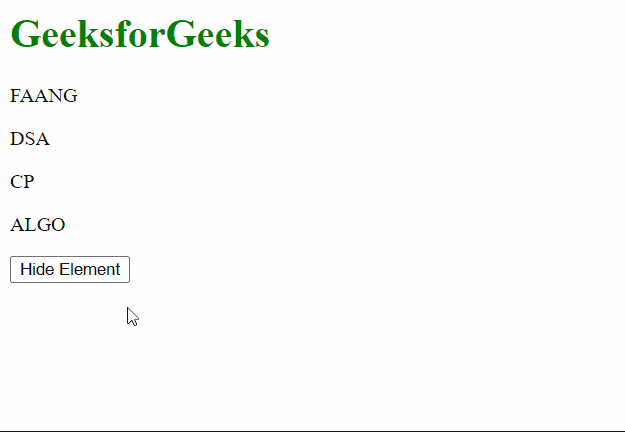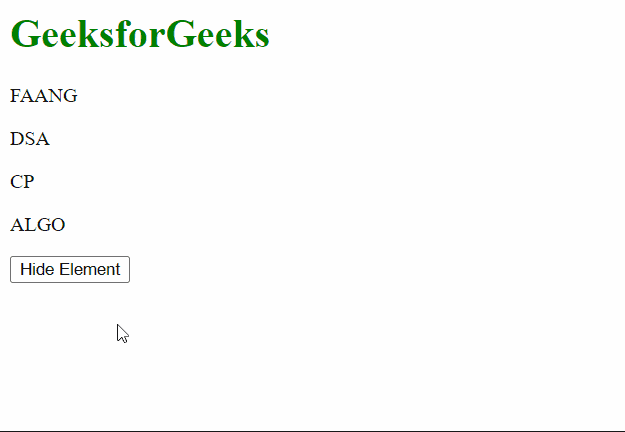# How to hide elements defined as variables in jQuery ?

• Last Updated : 07 Apr, 2021

In this article, we will learn how to hide elements defined as variables in jQuery. These can be done using two approaches.

Approach 1: In this approach, we will first select the element that has to be hidden and then assign it to a variable. We will then call the hide() method on the variable. This method will hide the element from the page.

Example:

## HTML

 `<``html``>``<``head``>``  ``<``script` `src``=``"https://code.jquery.com/jquery-3.6.0.js"``>``  ````  ``<``script``>``    ``\$(document).ready(function () {``      ``\$("button").click(function () {`` ` `        ``// Getting the element with the id``        ``// of "dsa" in a variable``        ``let dsaGFG = \$("#dsa");`` ` `        ``// Hiding the element using the``        ``// hide() method``        ``dsaGFG.hide();``      ``})``    ``});``  `````` ` `<``body``>``  ``<``h1` `style``=``"color: green;"``>``    ``GeeksforGeeks``  ````   ` `  ``<``p` `id``=``"faang"``>FAANG`` ` `  ``<``p` `id``=``"dsa"``>DSA`` ` `  ``<``p` `id``=``"cp"``>CP`` ` `  ``<``p` `id``=``"algo"``>ALGO`` ` `  ``<``button``>Hide Element`````

Output:Approach 2: In this approach, we will first select the element that has to be hidden and then assign it to a variable. We will then call the addClass() method on the variable. This will add a CSS class that we will create next. This CSS class will contain the display property that is set to none, effectively hiding the element.

Example:

## HTML

 `<``html``>``<``head``>``  ``<``style``>``    ``/* Define the class to``       ``be added */``    ``.hiddenClass {`` ` `      ``/* Setting the display``         ``to none hides the element */``      ``display: none;``    ``}``  ````  ``<``script` `src``=``"https://code.jquery.com/jquery-3.6.0.js"``>``  ````  ``<``script``>``    ``\$(document).ready(function () {``      ``\$("button").click(function () {`` ` `        ``// Getting the element with the id``        ``// of "cp" in a variable``        ``let cpGFG = \$("#cp");`` ` `        ``// Hiding the element by adding a``        ``// class using the addClass() method``        ``cpGFG.addClass("hiddenClass");``      ``})``    ``});``  ``````<``body``>``  ``<``h1` `style``=``"color: green;"``>``    ``GeeksforGeeks``  ````   ` `  ``<``p` `id``=``"faang"``>FAANG`` ` `  ``<``p` `id``=``"dsa"``>DSA`` ` `  ``<``p` `id``=``"cp"``>CP`` ` `  ``<``p` `id``=``"algo"``>ALGO`` ` `  ``<``button``>Hide Element`````

Output:My Personal Notes arrow_drop_up